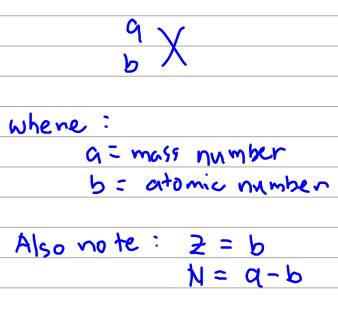# Problem: Which of the following isotopes would you expect to be stable?a. uranium-238b. 4Hec. oxygen-16d. 208Poe. 58Ni

###### FREE Expert Solution
82% (154 ratings)
###### FREE Expert Solution

We can know the stability of an isotope depending on the ratio of neutrons to protons but these criteria changes depending on the number of protons it has. We have listed below the ranges for the number of protons (Z) and the corresponding ratio (N/Z, where N is the number of neutrons) where the isotope would be most stable. The actual ratio doesn't have to be exact. It just has to be near the ideal ratio.Now we go through each choice. We are going to use the notation below to write the isotopes:82% (154 ratings)###### Problem Details

Which of the following isotopes would you expect to be stable?

a. uranium-238

b. 4He

c. oxygen-16

d. 208Po

e. 58Ni

What scientific concept do you need to know in order to solve this problem?

Our tutors have indicated that to solve this problem you will need to apply the Band of Stability concept. You can view video lessons to learn Band of Stability. Or if you need more Band of Stability practice, you can also practice Band of Stability practice problems.

What is the difficulty of this problem?

Our tutors rated the difficulty ofWhich of the following isotopes would you expect to be stabl...as high difficulty.

How long does this problem take to solve?

Our expert Chemistry tutor, Dasha took 3 minutes and 57 seconds to solve this problem. You can follow their steps in the video explanation above.

What professor is this problem relevant for?

Based on our data, we think this problem is relevant for Professor West's class at Southwest Baptist University.